Mobile QR Code1. (School of Electrical and Electronics Engineering, Chung-Ang University, Seoul 06974, Korea)
2. (Research and Development Center, Samsung Display, Yongin 17113, Korea)

Indium-gallium-zinc oxide (IGZO), thin-film transistors (TFTs), oxygen content, output curve, high current driving condition

## I. INTRODUCTION

Presently, indium-gallium-zinc oxide (IGZO) thin-film transistors (TFTs) are attracting significant attention because of their outstanding properties such as high electron mobility, excellent on/off ratio, high uniformity, and low process temperature [1-5]. Because of these merits, IGZO TFTs are being widely used as a backplane in large-area organic light-emitting diode (OLED) displays [6-9]. For the utilization of the IGZO TFTs for not only present OLED displays but also future OLED displays with higher brightness and resolution, TFTs should exhibit excellent electrical stability, particularly under high current driving conditions. Thus far, various studies have been conducted on the high-current stress-induced instability of IGZO TFTs [10-14]. The degradation of output characteristics under high-current operation conditions could be an important problem that alters all the characteristics of the systems comprising the TFTs. However, most previous studies evaluated the electrical stability of the IGZO TFTs under high current stresses using only their transfer characteristics, such as the threshold voltage (V$_{\mathrm{TH}}$) and subthreshold swing (SS). In this study, we comprehensively investigated the effects of oxygen content in the IGZO channel on the output characteristics of IGZO TFTs under high-current operation conditions using commercially available top-gate self-aligned (TG-SA) coplanar IGZO TFTs prepared with different oxygen partial pressures.

## II. EXPERIMENTAL DETAILS

The TG-SA coplanar IGZO TFTs with different oxygen contents within the channel layer were fabricated using the following process. First, a Mo layer was deposited on a glass substrate and patterned to form the bottom gate electrode (i.e., a light shield). Next, as a buffer layer, a SiO$_{\mathrm{X}}$ layer was formed using plasma-enhanced chemical vapor deposition (PECVD). IGZO films (In:Ga:Zn = 1:1:1: at \%) with different oxygen contents (oxygen-rich and oxygen-poor) were deposited by radio-frequency magnetron sputtering using a ceramic target at different oxygen partial pressures. A SiO$_{\mathrm{X}}$ film was deposited as a gate dielectric by PECVD. Subsequently, a Mo metal gate electrode was deposited. After the Mo gate electrode and SiO$_{\mathrm{X}}$ gate dielectric were deposited and patterned, PECVD was employed to deposit a SiO$_{\mathrm{X}}$ layer and a SiN$_{\mathrm{X}}$ layer as an interlayer dielectric (ILD), which was then patterned to form via holes. Source and drain electrodes of Al were deposited and patterned on the n+-IGZO source/drain extension regions. Finally, the devices were thermally annealed at 340 $^{\circ}$C to achieve stable and uniform electrical performances. The fabricated devices are schematically illustrated in Fig. 1. The output and transfer characteristics of the IGZO TFTs were measured using an Agilent 4156C parameter analyzer and capacitance-voltage (C-V) curves were obtained using an LCR meter (HP4284A). All measurements were performed at room temperature in a dark chamber.

##### Fig. 1. Schematic illustration of the fabricated TG-SA coplanar IGZO TFTs.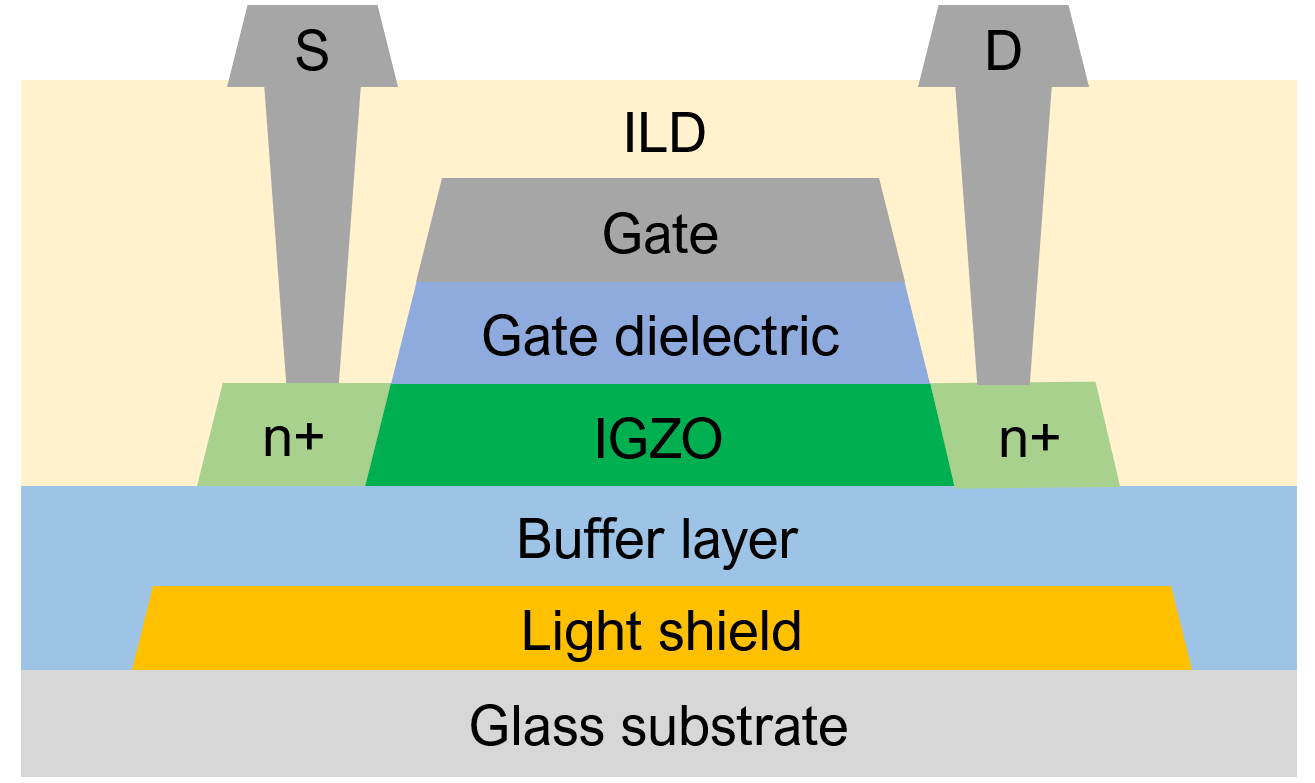## III. RESULTS AND DISCUSSION

Fig. 2(a) and (b) depict the output characteristics of oxygen-rich and oxygen-poor IGZO TFTs (width/length (W/L) = 3 ${\mu}$m/5 ${\mu}$m), respectively, measured under high-current operation conditions (gate-to-source voltage (V$_{\mathrm{GS}}$)) = 40 V). Fig. 2(a) and (b) show that the drain current (I$_{\mathrm{D}}$) gradually increases with an increase in V$_{\mathrm{DS}}$ at V$_{\mathrm{DS}}$ {\textless} \textasciitilde{} 20 V in both TFTs. However, it decreases as V$_{\mathrm{DS}}$ increases at V$_{\mathrm{DS}}$ > \textasciitilde{} 20 V in the oxygen-rich TFTs but abruptly increases with an increase in V$_{\mathrm{DS}}$ at V$_{\mathrm{DS}}$ > \textasciitilde{} 20 V in the oxygen-poor TFTs. The obtained results shown in Fig. 2(a) and (b) reveal that the amount of oxygen in the IGZO channel strongly affects the output characteristics of IGZO TFTs under high-current operation conditions. Fig. 2(c) and (d) show the transfer curves obtained before and after the characterization of output curves from oxygen-rich and oxygen-poor IGZO TFTs, respectively, at a drain-to-source voltage (V$_{\mathrm{DS}}$) of 0.1 V. As observed in Fig. 2(c) and (d), the transfer curves shift in the positive direction after output curve measurements in both TFTs; however, the degree of the transfer curve shift exhibits larger values in the oxygen-rich TFT, and the degradation of SS is more significant in the transfer curve of the oxygen-poor TFT after output curve characterization. Generally, the increase in V$_{\mathrm{TH}}$ reduces I$_{\mathrm{D}}$ in n-type TFTs; therefore, the result in Fig. 2(c) appears to be consistent with that in Fig. 2(a). However, an abrupt increase in I$_{\mathrm{D}}$ with V$_{\mathrm{DS}}$ observed in the oxygen-poor TFT (Fig. 2(b)) appears inconsistent with the results in Fig. 2(d) at first glance; therefore, further study is needed to elucidate the mechanism behind the abrupt increase in I$_{\mathrm{D}}$ with an increase in V$_{\mathrm{DS}}$ observed in the oxygen-poor TFT.

To reveal the physical mechanisms responsible for the phenomena observed in Fig. 2, we compared the transfer curves and C-V curves measured after sweeping V$_{\mathrm{DS}}$ up to V$_{\mathrm{DS,crt}}$ and V$_{\mathrm{DS.max}}$ in oxygen-rich and oxygen-poor IGZO TFTs, respectively. Here, V$_{\mathrm{DS,crt}}$ denotes the V$_{\mathrm{DS}}$ value at which I$_{\mathrm{D}}$ begins to decrease (in oxygen-rich TFTs) or abruptly increase (in oxygen-poor TFTs) and V$_{\mathrm{DS,max}}$ represents the maximum value of the sweep V$_{\mathrm{DS}}$ as depicted in Fig. 3(a) and (b). Fig. 3(c) and (d) show the transfer curves measured before sweeping V$_{\mathrm{DS}}$, after sweeping V$_{\mathrm{DS}}$ up to V$_{\mathrm{DS,crt}}$, and after sweeping V$_{\mathrm{DS}}$ up to V$_{\mathrm{DS,max}}$ from the oxygen-rich and oxygen-poor IGZO TFTs, respectively. Further, Fig. 3(c) reveals that the positive shift of the transfer curve becomes more significant as the value of the sweep V$_{\mathrm{DS}}$increases in the oxygen-rich IGZO TFT; however, the degree of the transfer curve shift becomes smaller, and the degradation of SS becomes more significant as the sweep V$_{\mathrm{DS}}$increases from V$_{\mathrm{DS,crt}}$ to V$_{\mathrm{DS,max}}$ in the oxygen-poor IGZO TFT. Fig. 3(e) and (f) show the small-signal C-V curves measured before sweeping V$_{\mathrm{DS}}$, after sweeping V$_{\mathrm{DS}}$ up to V$_{\mathrm{DS,crt}}$, and after sweeping V$_{\mathrm{DS}}$ up to V$_{\mathrm{DS,max}}$ from the oxygen-rich and oxygen-poor IGZO TFTs, respectively. Here, C-V curves were obtained between the gate and source/drain tied to the ground (i. e. C$_{\mathrm{GDS}}$-V$_{\mathrm{GS}}$) at a frequency of 100 kHz. Fig. 3(e) shows that the C$_{\mathrm{GDS}}$-V$_{\mathrm{GS}}$ curve shifts in the positive direction without significant distortion of the curve shape after characterization of the output curves and the degree of the positive shift increases as the value of the sweep V$_{\mathrm{DS}}$increases in the oxygen-rich IGZO TFT. However, the stretch-out of the C$_{\mathrm{GDS}}$-V$_{\mathrm{GS}}$ curve is observed after output curve characterization in the oxygen-poor IGZO TFTs, and it becomes more significant as the sweep V$_{\mathrm{DS}}$value increases from V$_{\mathrm{DS,crt}}$ to V$_{\mathrm{DS,max}}$. Furthermore, a significant decrease in the turn-on voltage of the C$_{\mathrm{GDS}}$-V$_{\mathrm{GS}}$ curve is observed after sweeping V$_{\mathrm{DS}}$ up to V$_{\mathrm{DS,max}}$.

The experimental results in Fig. 3 clearly show that the output curve sweep under the high-V$_{\mathrm{GS}}$ condition affects the electrical characteristics of IGZO TFTs in a different manner depending on the amount of oxygen within the IGZO channel layer and sweep V$_{\mathrm{DS}}$ value. Fig. 3(c) and (e) reveal that the transfer curve and C$_{\mathrm{GDS}}$-V$_{\mathrm{GS}}$ curve further shift in the positive direction as the sweep V$_{\mathrm{DS}}$ value increases from V$_{\mathrm{DS.crt}}$ to V$_{\mathrm{DS.max}}$, without significant curve distortion in the oxygen-rich IGZO TFT. Considering that the electron trapping into the gate dielectric parallelly shifts the transfer and C$_{\mathrm{GDS}}$-V$_{\mathrm{GS}}$ curves in the positive direction in IGZO TFTs [15-18], it implies that the number of trapped electrons within the gate dielectric further increases with an increase in the sweep V$_{\mathrm{DS}}$ value during the output curve characterization. The increase in the sweep V$_{\mathrm{DS}}$ value increases I$_{\mathrm{D}}$ and device temperature momentarily , which facilitates the electron trapping into the gate dielectric under the high-V$_{\mathrm{GS}}$ condition [20-22], consequently increasing V$_{\mathrm{TH}}$. The increase in V$_{\mathrm{TH}}$ reduces I$_{\mathrm{D}}$, which explains the decrease in I$_{\mathrm{D}}$ at V$_{\mathrm{DS}}$ > V$_{\mathrm{DS,crt}}$ from the oxygen-rich IGZO TFT observed in Fig. 2(a). Further, Fig. 3(d) and (f) reveal that the transfer curve and C$_{\mathrm{GDS}}$-V$_{\mathrm{GS}}$ curve shift in the negative direction as the sweep V$_{\mathrm{DS}}$ value increases from V$_{\mathrm{DS.crt}}$ to V$_{\mathrm{DS.max}}$ with a significant curve distortion in the oxygen-poor IGZO TFT, which shows that the positively charged subgap states are generated during the V$_{\mathrm{DS}}$ sweep from V$_{\mathrm{DS.crt}}$ to V$_{\mathrm{DS.max}}$ at high V$_{\mathrm{GS}}$ (= 40 V).

Because the defect formation energy for oxygen vacancies (V$_{\mathrm{O}}$) in the oxygen-poor IGZO is much smaller than that in the oxygen-rich IGZO , the generated positively charged subgap states are considered doubly ionized oxygen vacancies (V$_{\mathrm{O}}$$^{2+}) acting as shallow donors in IGZO . The decrease in V_{\mathrm{TH}} increases I_{\mathrm{D}}, which explains the abrupt increase in I_{\mathrm{D}} at V_{\mathrm{DS}} > V_{\mathrm{DS,crt}} from the oxygen-poor IGZO TFT observed in Fig. 2(b). Fig. 4 shows the schematic illustration of the trapped electrons and generated V_{\mathrm{O}}$$^{2+}$ states in oxygen-rich and oxygen-poor IGZO TFTs after V$_{\mathrm{DS}}$ sweeping to V$_{\mathrm{DS.crt}}$ and V$_{\mathrm{DS.max}}$, respectively, at high V$_{\mathrm{GS}}$. The increase in the number of trapped electrons within the gate dielectric is more significant than the increase in the number of V$_{\mathrm{O}}$$^{2+} states after increasing the sweep V_{\mathrm{DS}} value to V_{\mathrm{DS.crt}} and V_{\mathrm{DS.max}} in the oxygen- rich IGZO TFTs; however, the increase in the number of V_{\mathrm{O}}$$^{2+}$ states is more significant than that of the trapped electrons within the gate dielectric in the oxygen-poor IGZO TFTs.

Fig. 5(a) and (b) depict the output characteristics of oxygen-rich and oxygen-poor IGZO TFTs, respectively, with different Ws (W/L = 2.5 ${\mu}$m/5 ${\mu}$m, 3 ${\mu}$m/5 ${\mu}$m, and 5 ${\mu}$m/5 ${\mu}$m) measured under high-current operation conditions. Fig. 5(a) shows that I$_{\mathrm{D}}$ decreases with V$_{\mathrm{DS}}$ at V$_{\mathrm{DS}}$ > V$_{\mathrm{DS,crt}}$ in the oxygen-rich IGZO TFTs with each W; however, the I$_{\mathrm{D}}$ decrease becomes more significant as W increases. Moreover, Fig. 5(b) shows that I$_{\mathrm{D}}$ gradually increases with V$_{\mathrm{DS}}$ in oxygen-poor IGZO TFTs with W = 2.5 and 3 ${\mu}$m; however, it abruptly increases with V$_{\mathrm{DS}}$ at V$_{\mathrm{DS}}$ > V$_{\mathrm{DS,crt}}$ in the TFT with W = 5 ${\mu}$m. Fig. 5(c) and (d) show the W-normalized C$_{\mathrm{GDS}}$-V$_{\mathrm{GS}}$ curves measured before and after sweeping V$_{\mathrm{DS}}$ from the oxygen-rich and oxygen-poor IGZO TFTs, respectively, with every dimension. Fig. 5(c) shows that the W-normalized C$_{\mathrm{GDS}}$-V$_{\mathrm{GS}}$ curve obtained from oxygen-rich IGZO TFTs with each W shifts in the positive direction after V$_{\mathrm{DS}}$ sweep; however, the degree of the transfer curve shift becomes more significant as the W of the IGZO TFT increases. Meanwhile, Fig. 5(d) shows that the W-normalized C$_{\mathrm{GDS}}$- V$_{\mathrm{GS}}$ curves measured from the oxygen-poor IGZO TFTs with W = 2.5 and 3 ${\mu}$m are only slightly distorted after V$_{\mathrm{DS}}$ sweep; however, a significant stretch-out of the W-normalized C$_{\mathrm{GDS}}$-V$_{\mathrm{GS}}$ curve is observed with a marked decrease in the turn-on voltage in the oxygen-poor IGZO TFTs with W = 5 ${\mu}$m after V$_{\mathrm{DS}}$ sweep. The experimental results in Fig. 5 clearly show that the output curve degradation at high V$_{\mathrm{DS}}$s under high-current operation conditions becomes more significant as W increases in both oxygen-rich and oxygen-poor IGZO TFTs. Considering that the self-heating effect is enhanced in the IGZO TFT with a higher channel width because of the low thermal conductivity of IGZO [25,26], the results in Fig. 5 confirm that the abnormal behavior of the output curves mainly due to the electron trapping into the gate dielectric in the oxygen-rich IGZO TFT and the V$_{\mathrm{O}}$$^{2+} state generation in the oxygen-poor IGZO TFT are accelerated by the increased thermal energy due to the higher device temperature. ##### Fig. 2. Output curves measured from (a) oxygen-rich; (b) oxygen-poor IGZO TFTs with W/L = 3 {\mu}m/5 {\mu}m under high-current operation conditions (V_{\mathrm{GS}} = 40 V). Transfer curves measured from (c) oxygen-rich; (d) oxygen-poor IGZO TFTs before and after the characterization of output curves at V_{\mathrm{DS}} = 0.1 V.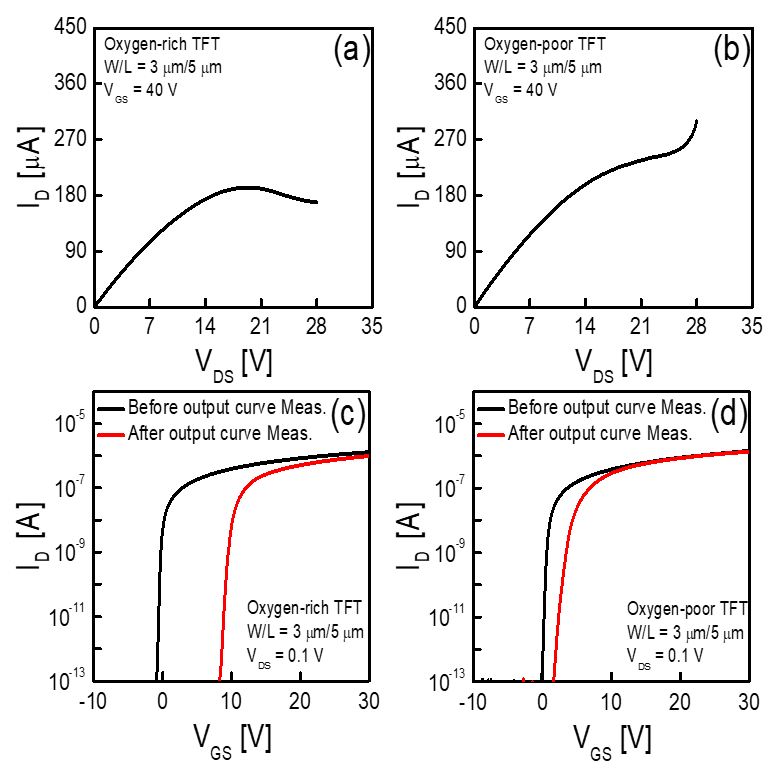##### Fig. 3. Output curves measured from (a) oxygen-rich; (b) oxygen-poor IGZO TFTs with different V_{\mathrm{DS}} sweeps ranging from 0 (V) to V_{\mathrm{DS,crt}} (V) and 0 (V) to V_{\mathrm{DS,max}} (V), where V_{\mathrm{DS,crt}} denotes the V_{\mathrm{DS}} value at which I_{\mathrm{D}} begins to decrease (in oxygen-rich TFTs) or abruptly increase (in oxygen-poor TFTs) and V_{\mathrm{DS,max}} represents the maximum value of the sweep V_{\mathrm{DS}}. Transfer curves measured from (c) oxygen-rich; (d) oxygen-poor IGZO TFTs before sweeping V_{\mathrm{DS}}, after sweeping V_{\mathrm{DS}} up to V_{\mathrm{DS,crt}}, and after sweeping V_{\mathrm{DS}} up to V_{\mathrm{DS,max}} at V_{\mathrm{DS}} = 0.1 V. C_{\mathrm{GDS}}-V_{\mathrm{GS}}curves measured from (e) oxygen-rich; (f) oxygen-poor IGZO TFTs before sweeping V_{\mathrm{DS}}, after sweeping V_{\mathrm{DS}} up to V_{\mathrm{DS,crt}}, and after sweeping V_{\mathrm{DS}} up to V_{\mathrm{DS,max}} at a frequency of 100 kHz.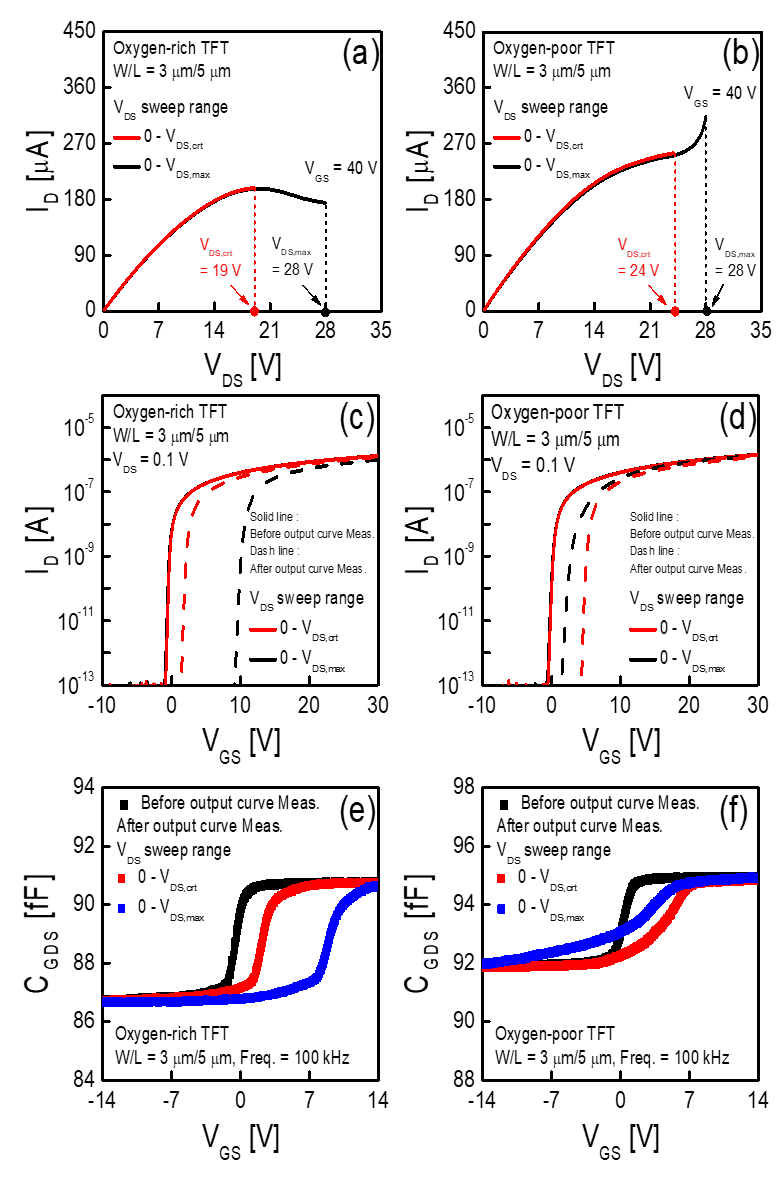##### Fig. 4. Schematic illustration of the trapped electrons in the gate dielectric and generated V_{\mathrm{O}}$$^{2+}$ states in IGZO in oxygen-rich and oxygen-poor IGZO TFTs after V$_{\mathrm{DS}}$ sweep to V$_{\mathrm{DS.crt}}$ and V$_{\mathrm{DS.max}}$ at high V$_{\mathrm{GS}}$.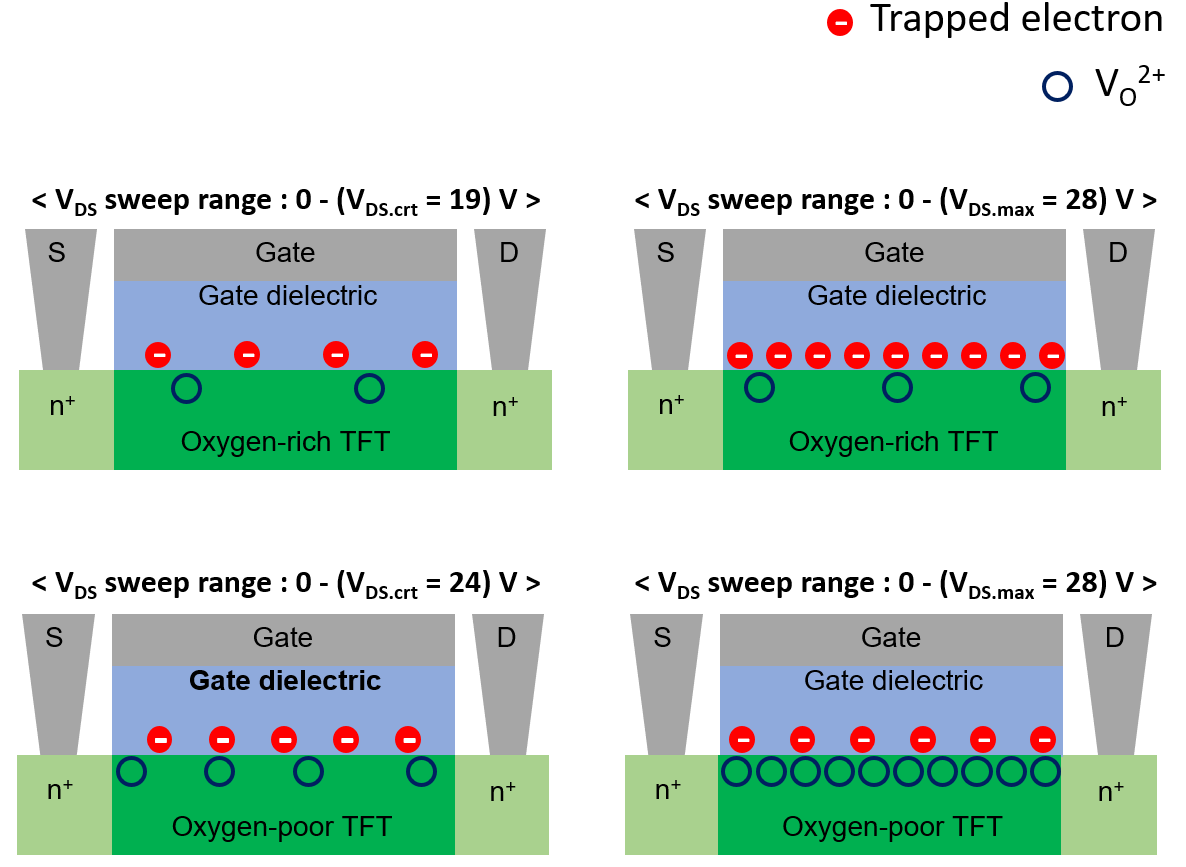##### Fig. 5. Output curves measured from (a) oxygen-rich; (b) oxygen-poor IGZO TFTs with different Ws (W/L = 2.5~${\mu}$m/5~${\mu}$m, 3 ${\mu}$m/5 ${\mu}$m, and 5 ${\mu}$m/5 ${\mu}$m) under high-current operation conditions. W-normalized C$_{\mathrm{GDS}}$-V$_{\mathrm{GS}}$ curves measured from (c) oxygen-rich; (d) oxygen-poor IGZO TFTs with different Ws (W/L = 2.5 ${\mu}$m/5 ${\mu}$m, 3 ${\mu}$m/5 ${\mu}$m, 5 ${\mu}$m/5 ${\mu}$m) before and after the characterization of output curves at a frequency of 100 kHz.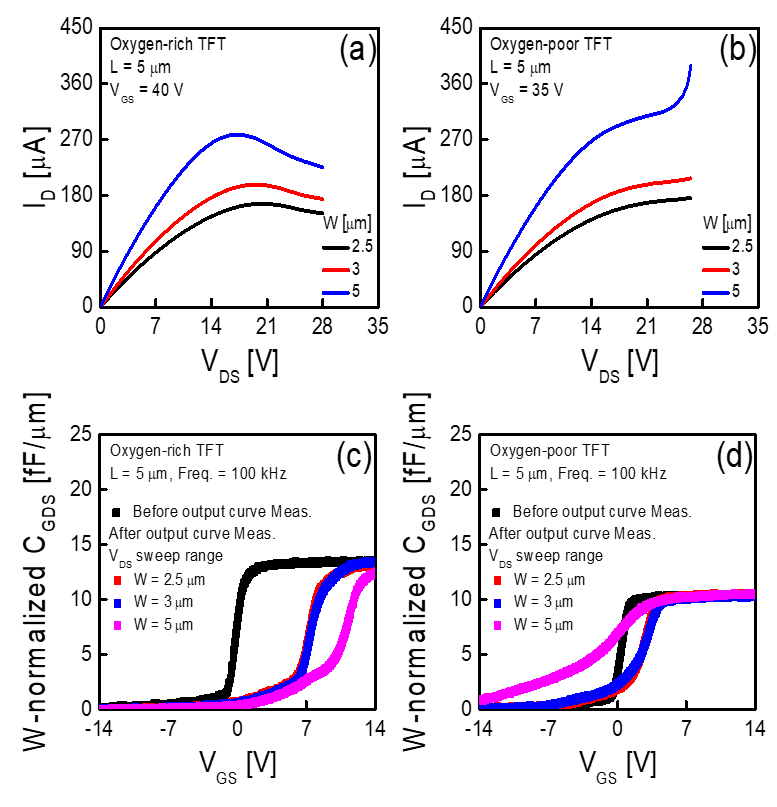## IV. CONCLUSIONS

In this study, we examine the effects of oxygen content in the IGZO channel on the output characteristics of IGZO TFTs under high-current operation conditions. Output curves were measured from both oxygen-rich and oxygen-poor IGZO TFTs under high-current conditions (at V$_{\mathrm{GS}}$ = 40 V), and the characterization results showed that I$_{\mathrm{D}}$ decreased with V$_{\mathrm{DS}}$at V$_{\mathrm{DS}}$ > V$_{\mathrm{DS,crt}}$ in the oxygen-rich IGZO TFT; however, it abruptly increased with V$_{\mathrm{DS}}$at V$_{\mathrm{DS}}$ > V$_{\mathrm{DS,crt}}$ in the oxygen-rich IGZO TFT. The transfer and C$_{\mathrm{GDS}}$-V$_{\mathrm{GS}}$ curves measured after sweeping V$_{\mathrm{DS}}$ up to V$_{\mathrm{DS,crt}}$ and V$_{\mathrm{DS.max}}$ in the oxygen-rich IGZO TFTs, respectively, revealed that the positive shift of the transfer and C$_{\mathrm{GDS}}$-V$_{\mathrm{GS}}$ curves became more significant as the value of the sweep V$_{\mathrm{DS}}$increases; therefore, the decrease in I$_{\mathrm{D}}$ with V$_{\mathrm{DS}}$at V$_{\mathrm{DS}}$ > V$_{\mathrm{DS,crt}}$ in the oxygen-rich IGZO TFT is mainly attributed to the enhanced electron trapping into the gate dielectric with an increase in sweep V$_{\mathrm{DS}}$. However, the transfer and C$_{\mathrm{GDS}}$-V$_{\mathrm{GS}}$ curves shifted in the negative direction with a distortion of the curve shape when the sweep V$_{\mathrm{DS}}$increased from V$_{\mathrm{DS,crt}}$ to V$_{\mathrm{DS,max}}$ in the oxygen-poor IGZO TFT, implying that the abrupt increase in I$_{\mathrm{D}}$ with V$_{\mathrm{DS}}$at V$_{\mathrm{DS}}$ > V$_{\mathrm{DS,crt}}$ in the oxygen-poor IGZO TFT is possibly attributed to the enhanced V$_{\mathrm{O}}$$^{2+}$ generation with an increase in sweep V$_{\mathrm{DS}}$ considering that the defect formation energy for V$_{\mathrm{O}}$ is smaller in the oxygen-poor IGZO than in the oxygen-rich IGZO. The abnormal behavior of the output curves at high V$_{\mathrm{DS}}$s under high-current operation conditions becomes more significant as W increases in both oxygen-rich and oxygen-poor IGZO TFTs, which is ascribed to the increased device temperature due to the self-heating effects.

## ACKNOWLEDGMENTS

This research was supported by the Chung-Ang University Research Scholarship Grants in 2021, Samsung Display Co., Ltd., and the National Research Foundation of Korea (NRF) grant funded by the Korean government (MSIT) (2020R1A2B5B01001765).

## References

1
T. Kamiya et al., “Present status of amorphous In-Ga-Zn-O thin-film transistors,” Science and Technology of Advanced Materials, Vol. 11, No. 4, Nov., 2010.2
A. Nathan et al., “Transparent oxide semicon-ductors for advanced display applications,” Information Display, Vol. 29, No. 1, pp. 6-11, Jan., 2013.3
T. Kamiya et al., “Origins of High Mobility and Low Operation Voltage of Amorphous Oxide TFTs: Electronic Structure, Electron Transport, Defects and Doping,” Journal of Display Technology, Vol. 5, No. 7, pp. 273-288, Dec., 2009.4
K. Nomura et al., “Room-temperature fabrication of transparent flexible thin-film transistors using amorphous oxide semiconductors,” Nature, Vol. 432, No. 7016, pp. 488-492, Nov., 2004.5
K. Nomura et al., “Amorphous oxide semiconductors for high-performance flexible thin-film transistors,” Japanese Journal of Applied Physics, Vol. 45, No. 5B, pp. 4303-4308, May, 2006.6
H. Hsieh et al., “Development of IGZO TFTs and their applications to next-generation flat-panel displays,” Journal of Information Display, Vol. 11, No. 4, pp. 160-164, Dec., 2010.7
H. N. Lee et al., “Oxide TFT with multilayer gate insulator for backplane of AMOLED device,” Journal of the Society for Information Display, Vol. 16, No. 2, pp. 265-272, Feb., 2008.8
J. Park et al., “Effect of positive bias stress on the back-gate voltage-modulated threshold voltage in double-gate amorphous InGaZnO thin-film transistors,” IEEE Electron Device Letters, Vol. 43, No. 11, pp. 1878-1881, Nov., 2022.9
A. Sodhani et al., “Design of threshold voltage insensitive pixel driver circuitry using a-IGZO TFT for AMOLED displays,” Microelectronics Journal, Vol. 101, pp. 104819, Jul., 2020.10
A. Sodhani et al., “Design of threshold voltage insensitive pixel driver circuitry using a-IGZO TFT for AMOLED displays,” Microelectronics Journal, Vol. 101, pp. 104819, Jul., 2020.11
H. Yang et al., “Self-Heating Stress-Induced Severe Humps in Transfer Characteristics of Amorphous InGaZnO Thin-Film Transistors,” IEEE Transactions on Electron Devices, Vol. 68, No. 12, pp. 6197-3201, Dec., 2021.12
S. Choi et al., “Effect of oxygen content on current stress-induced instability in bottom-gate amorphous InGaZnO thin-film transistors,” Materials, Vol. 12, No. 19, pp. 3149, Sep., 2019.13
M. Mativenga et al., “High current stress effects in amorphous-InGaZnO4 thin-film transistors,” Applied Physics Letters, Vol. 102, No. 2, pp. 023503, Dec., 2013.14
W. S. Kim et al., “Abnormal behavior with hump characteristics in current stressed a-InGaZnO thin film transistors,” Solid-State Electronics, Vol. 137, pp. 22-28, Nov., 2017.15
Y. S. Lee et al., “Oxygen flow effects on electrical properties, stability, and density of states of amorphous In-Ga-Zn-O thin-film transistors,” Japanese Journal of Applied Physics, Vol. 53, No. 12, pp. 121101, Nov., 2014.16
S. Choi et al., “Effect of channel widths on negative shift of threshold voltage, including stress-induced hump phenomenon in InGaZnO thin-film transistors under high-gate and drain bias stress,” Applied Physics Letters, Vol. 100, No. 4, pp. 043503, Jan., 2012.17
T. Hsieh et al., “Investigation of gate-bias stress and hot-carrier stress-induced instability of InGaZnO thin-film transistors under different environments,” Surface and Coatings Technology, Vol. 231, pp. 478-481, Sep., 2013.18
C. Kuo et al., “Abnormal Subthreshold Swing Decrease in a-InGaZnO Thin-Film Transistor After Self-Heating Stress,” IEEE Transactions on Electron Devices, pp. 1-5, Nov., 2022.19
S. W. Lee et al., “Abnormal Output Characteristics of p-Type Low Temperature Polycrystalline Silicon Thin Film Transistor Fabricated on Polyimide Substrate,” IEEE Journal of the Electron Devices Society, Vol. 4, No. 1, pp. 7-10, Jan., 2016.20
H. R. Yu et al., “Degradation on the Current Saturation of Output Characteristics in Amorphous InGaZnO Thin-Film Transistors,” IEEE Journal of the Electron Devices Society, Vol. 65, No. 8, pp. 3243-3249, Aug., 2018.21
T. Chen et al., “Self-heating enhanced charge trapping effect for InGaZnO thin film transistor,” Applied Physics Letters, Vol. 101, No. 4, pp. 042101, Jul., 2012.22
T. Hsieh et al., “Self-heating-effect-induced degradation behaviors in a-InGaZnO thin-film transistors,” IEEE Electron Device Letters, Vol. 34, No. 1, pp. 63-65, Nov., 2012.23
H. Li et al., “Oxygen vacancies and hydrogen in amorphous In-Ga-Zn-O and ZnO,” Physical Review Materials, Vol. 2, No. 7, pp. 074601, Jul., 2018.24
C. Y. Jeong et al., “A study on the degradation mechanism of InGaZnO thin-film transistors under simultaneous gate and drain bias stresses based on the electronic trap characterization,” Semiconductor Science and Technology, Vol. 29, No. 4, pp. 045023, Jul., 2014.25
T. Chen et al., “Self-heating enhanced charge trapping effect for InGaZnO thin film transistor,” Applied Physics Letters, Vol. 101, No. 4, pp. 042101, Feb., 2012.26
K. Liu et al., “Investigation of channel width-dependent threshold voltage variation in a-InGaZnO thin-film transistors,” Applied Physics Letters, Vol. 104, No. 13, pp. 133503, Feb., 2014Chae-Eun Oh received the B.S. degree in nano & semiconductor engineering from Korea Polytechnic University, Jeong-wang, South Korea, in 2021. She is currently pursuing the M.S. degree in electrical and electronics engineering from Chung-Ang University. Her current research interest includes reliability study of oxide thin-film transistors.

Hwan-Seok Jeong received the B.S. degree in chemistry from Dae-Jin University, Pochen, South Korea, in 2015. He is currently pursuing the Ph.D. degree in electrical and electronics engineering from Chung-Ang University. His current research interest includes the fabrication and reliability study of oxide thin-film transistors.

Su-Hyeon Lee received the B.S. degree in semiconductor physics from Korea University, Sejong, South Korea, in 2021. She is currently pursuing the M.S. degree in electrical and electronics engineering from Chung-Ang University. Her current research interest includes the fabrication and reliability study of oxide thin-film transistors.

Dong-Ho Lee received the B.S degree in electronic engineering from Gachon University, Gyeonggi-Do, South Korea, in 2020. Since 2020, He is currently pursuing the integrated M.S., Ph.D. degrees in electrical and electronics engineering from Chung-Ang University. His current research interest includes the reliability study of oxide thin-film transistors.

Yeong-Gil Kim received the B.S. degree in electronic engineering from Seoul National University of Science and Technology, Seoul, South Korea, in 2022. He is currently pursuing the M.S. degree in electrical and electronics engineering from Chung-Ang University. His current research interest includes the fabrication and reliability study of oxide thin-film transistors.

##### Myeong-Ho Kim

Myeong-Ho Kim is a research engineer with the Research and Development Center, Samsung Display, Yongin, South Korea.

##### Kyoung Seok Son

Kyoung Seok Son is a research engineer with the Research and Development Center, Samsung Display, Yongin, South Korea.

##### Jun Hyung Lim

Jun Hyung Lim received the Ph.D. degree from the Department of Materials Science and Engineering, Sungkyunkwan University, Suwon, South Korea, in 2006. He is in charge of the oxide backplane with the Research and Development Center, Samsung Display, Yongin, South Korea.

Sang-Hun Song received his BS degree in Electronics Engineering from Seoul National University in 1886 and his MA and Ph.D. degrees from Princeton University in 1988 and 1997, respectively. His doctoral research studies on magneto-optical and magneto-transport properties of the 2- dimensional carriers in strained semiconductor layers. In 1997, he joined LG Semicon Co. Ltd. As a DRAM circuit designer. In 2001, he joined the School of Electrical and Electronics Engineering at Chung-Ang University in Seoul, where his now a professor. His research interests include semiconductor materials and devices, and their applications to real world electronic systems.

Hyuck-In Kwon received the B.S., M.S., and Ph.D. degrees in electrical engineering from Seoul National University, Seoul, South Korea, in 1999, 2001, and 2005, respectively. From August 2004 to March 2006, he was a Research Associate with the University of Illinois at Urbana–Champaign. In 2006, he joined the System LSI Division, Samsung Electronics Company, South Korea, where he was a Senior Engineer with the Image Development Team. From September 2007 to February 2010, he was with the School of Electronic Engineering, Daegu University, as a full-time Lecturer and an Assistant Professor. Since 2010, he has been with Chung-Ang University, Seoul, where he is currently a Professor with the School of Electrical and Electronics Engineering. His research interests include CMOS active pixel image sensors, oxide thin-film transistors, and silicon nanotechnologies.